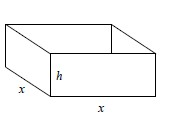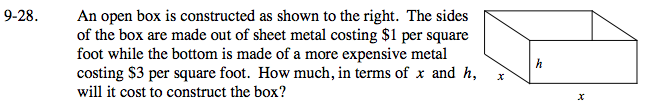### Home > PC > Chapter 9 > Lesson 9.1.2 > Problem9-28

9-28.
1. An open box is constructed as shown below. The sides of the box are made out of sheet metal costing $1 per square foot while the bottom is made of a more expensive metal costing$3 per square foot. How much, in terms of x and h, will it cost to construct the box? Homework Help ✎

2.1. Find the surface area.
2. Sum up the cost of each part.

$\text{Surface Area }= x^2 + 2xh + 2xh = x^2 + 4xh$

$\text{Cost of Construction }= (3\text{ dollars})x^2 + (1\text{ dollar}) 4xh = 3x^2 + 4xh$